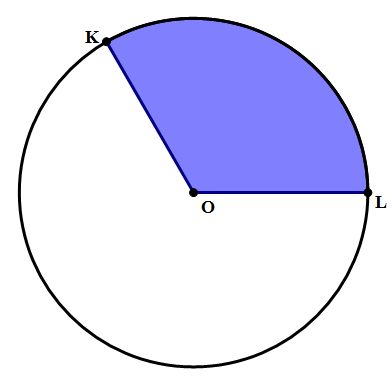Summer is Coming! Join the Game of Timers Competition to Win Epic Prizes. Registration is Open. Game starts Mon July 1st.

 It is currently 22 Jul 2019, 02:58### GMAT Club Daily Prep

#### Thank you for using the timer - this advanced tool can estimate your performance and suggest more practice questions. We have subscribed you to Daily Prep Questions via email.

Customized
for You

we will pick new questions that match your level based on your Timer History

Track

every week, we’ll send you an estimated GMAT score based on your performance

Practice
Pays

we will pick new questions that match your level based on your Timer History

#### Not interested in getting valuable practice questions and articles delivered to your email? No problem, unsubscribe here.# In the shaded region above, ∠KOL = 120°, and the area of the entire ci

Author Message
TAGS:

### Hide Tags

Math ExpertV
Joined: 02 Sep 2009
Posts: 56350
In the shaded region above, ∠KOL = 120°, and the area of the entire ci  [#permalink]

### Show Tags00:00

Difficulty:5% (low)

Question Stats:91% (01:28) correct9% (01:42) wrongbased on 64 sessions

### HideShow timer StatisticsIn the shaded region above, ∠KOL = 120°, and the area of the entire circle is $$A = 144\pi$$. The perimeter of the shaded region is

(A) 12 + 8{pi}
(B) 12 + 16{pi}
(C) 24 + 8{pi}
(D) 24 + 16{pi}
(E) 24 + 24{pi}

Kudos for a correct solution.

Attachment:c2_img2.png [ 9.13 KiB | Viewed 2168 times ]

_________________
InternJoined: 21 Sep 2013
Posts: 27
Location: United States
Concentration: Finance, General Management
GMAT Date: 10-25-2013
GPA: 3
WE: Operations (Mutual Funds and Brokerage)
Re: In the shaded region above, ∠KOL = 120°, and the area of the entire ci  [#permalink]

### Show Tags

1
Bunuel wrote:
In the shaded region above, ∠KOL = 120°, and the area of the entire circle is $$A = 144\pi$$. The perimeter of the shaded region is

(A) 12 + 8{pi}
(B) 12 + 16{pi}
(C) 24 + 8{pi}
(D) 24 + 16{pi}
(E) 24 + 24{pi}

Kudos for a correct solution.

pi r^2=144 pi
r=12

2sides of sector =24(2 radii of circle)
length of arc=Q/360*2pi*r
=120/360*2 pi r
=8 pi
thereforeperimitere is 24+8pi (c)
Senior ManagerB
Joined: 28 Feb 2014
Posts: 294
Location: United States
Concentration: Strategy, General Management
Re: In the shaded region above, ∠KOL = 120°, and the area of the entire ci  [#permalink]

### Show Tags

1
Bunuel wrote:
In the shaded region above, ∠KOL = 120°, and the area of the entire circle is $$A = 144\pi$$. The perimeter of the shaded region is

(A) 12 + 8{pi}
(B) 12 + 16{pi}
(C) 24 + 8{pi}
(D) 24 + 16{pi}
(E) 24 + 24{pi}

Kudos for a correct solution.

Here is the original image, courtesy of MagooshArea=144$${pi}$$, so radius =12

Perimeter of the shaded portion of the circle is C=(2*{pi}*12)/3 = 8$${pi}$$
8$${pi}$$+2 radii = 8$${pi}$$ + 24

SVPStatus: The Best Or Nothing
Joined: 27 Dec 2012
Posts: 1787
Location: India
Concentration: General Management, Technology
WE: Information Technology (Computer Software)
Re: In the shaded region above, ∠KOL = 120°, and the area of the entire ci  [#permalink]

### Show Tags

1
Answer (C) $$24 + 8{pi}$$

Refer diagram below

Attachment:c2_img2.png [ 14.21 KiB | Viewed 2126 times ]

$$\pi r^2 = 144\pi$$

Perimeter of the shaded curve (only) $$= \frac{2\pi*12}{3} = 8\pi$$

Total perimeter $$= 12+12+8\pi = 24+8\pi$$
_________________
Kindly press "+1 Kudos" to appreciateMath ExpertV
Joined: 02 Sep 2009
Posts: 56350
Re: In the shaded region above, ∠KOL = 120°, and the area of the entire ci  [#permalink]

### Show Tags

Bunuel wrote:In the shaded region above, ∠KOL = 120°, and the area of the entire circle is $$A = 144\pi$$. The perimeter of the shaded region is

(A) 12 + 8{pi}
(B) 12 + 16{pi}
(C) 24 + 8{pi}
(D) 24 + 16{pi}
(E) 24 + 24{pi}

Kudos for a correct solution.

Attachment:
c2_img2.png

MAGOOSH OFFICIAL SOLUTION:

The area $$144\pi=\pi{r^2}$$, so r = 12. This means KO = 12 and OL = 12, so those two sides together are 24. The remaining side is arc KL. The whole circumference is $$C=2\pi{r}=24\pi$$. The angle of 120° is 1/3 of a circle, so the arclength is 1/3 of the circumference. This means, $$arclength=8\pi$$, and therefore the entire perimeter is $$24 + 8\pi$$.

_________________
Non-Human UserJoined: 09 Sep 2013
Posts: 11724
Re: In the shaded region above, ∠KOL = 120°, and the area of the entire ci  [#permalink]

### Show Tags

Hello from the GMAT Club BumpBot!

Thanks to another GMAT Club member, I have just discovered this valuable topic, yet it had no discussion for over a year. I am now bumping it up - doing my job. I think you may find it valuable (esp those replies with Kudos).

Want to see all other topics I dig out? Follow me (click follow button on profile). You will receive a summary of all topics I bump in your profile area as well as via email.
_________________Re: In the shaded region above, ∠KOL = 120°, and the area of the entire ci   [#permalink] 13 Feb 2019, 23:03
Display posts from previous: Sort by

# In the shaded region above, ∠KOL = 120°, and the area of the entire ci